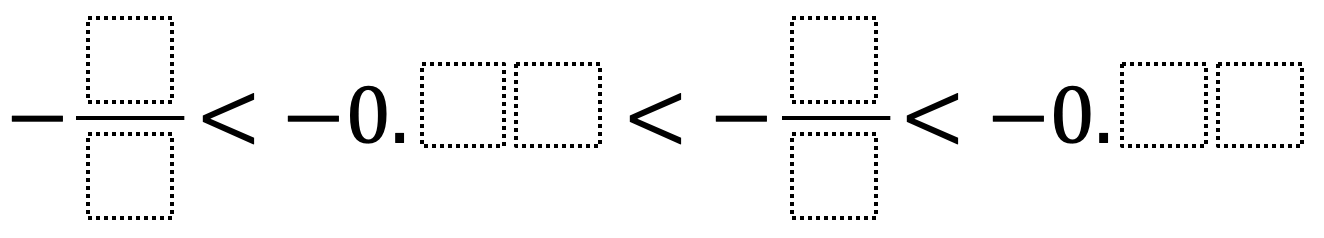# Ordering Rational Numbers

Directions: Using the digits 1 to 9 at most once each, fill in the boxes to make a true statement.### Hint

What importance does the relationship between the numerator and denominator have in determining the value of a fraction? What are some strategies to compare fractions and decimals?

There are various answers. A possible answer: -9/8 < -0.76 < -2/5 < -0.13 or -9/2 < -0.83 < -4/6 < -0.15

Source: Robert Millett

## Ratios 1

Directions: Using the digits 0 to 9 at most one time each, fill in the …

### One comment

1.A possible answer: -9/8 < -0.76 < -2/5 < -0.13 or -9/2 < -0.83 < -4/6 < -0.15